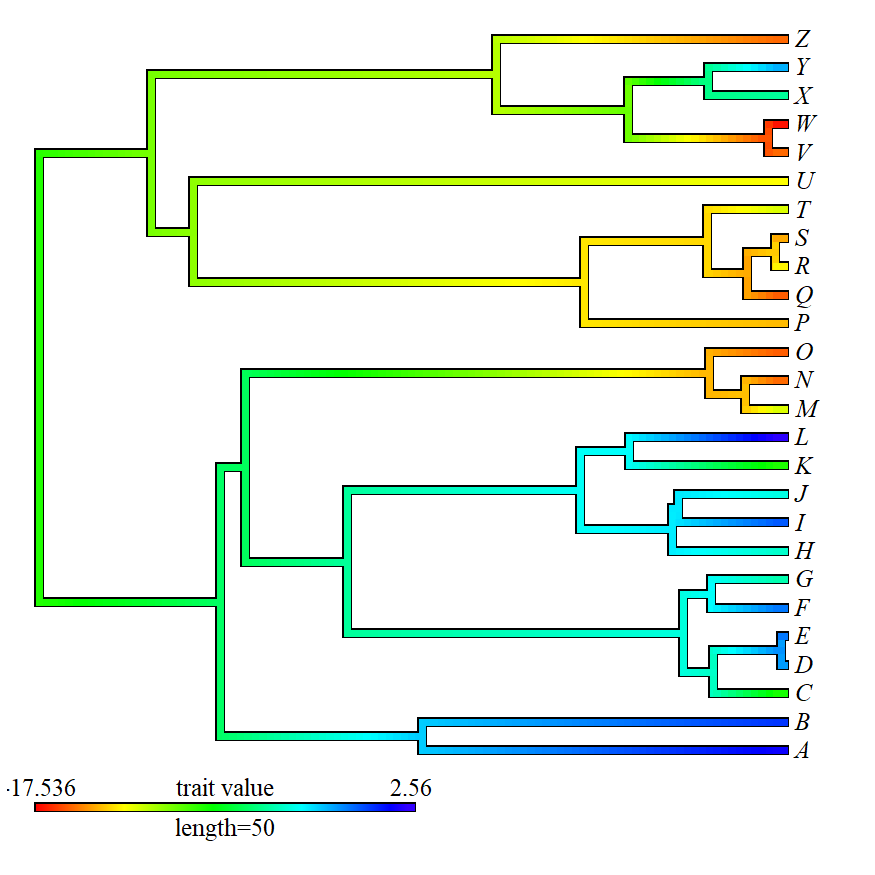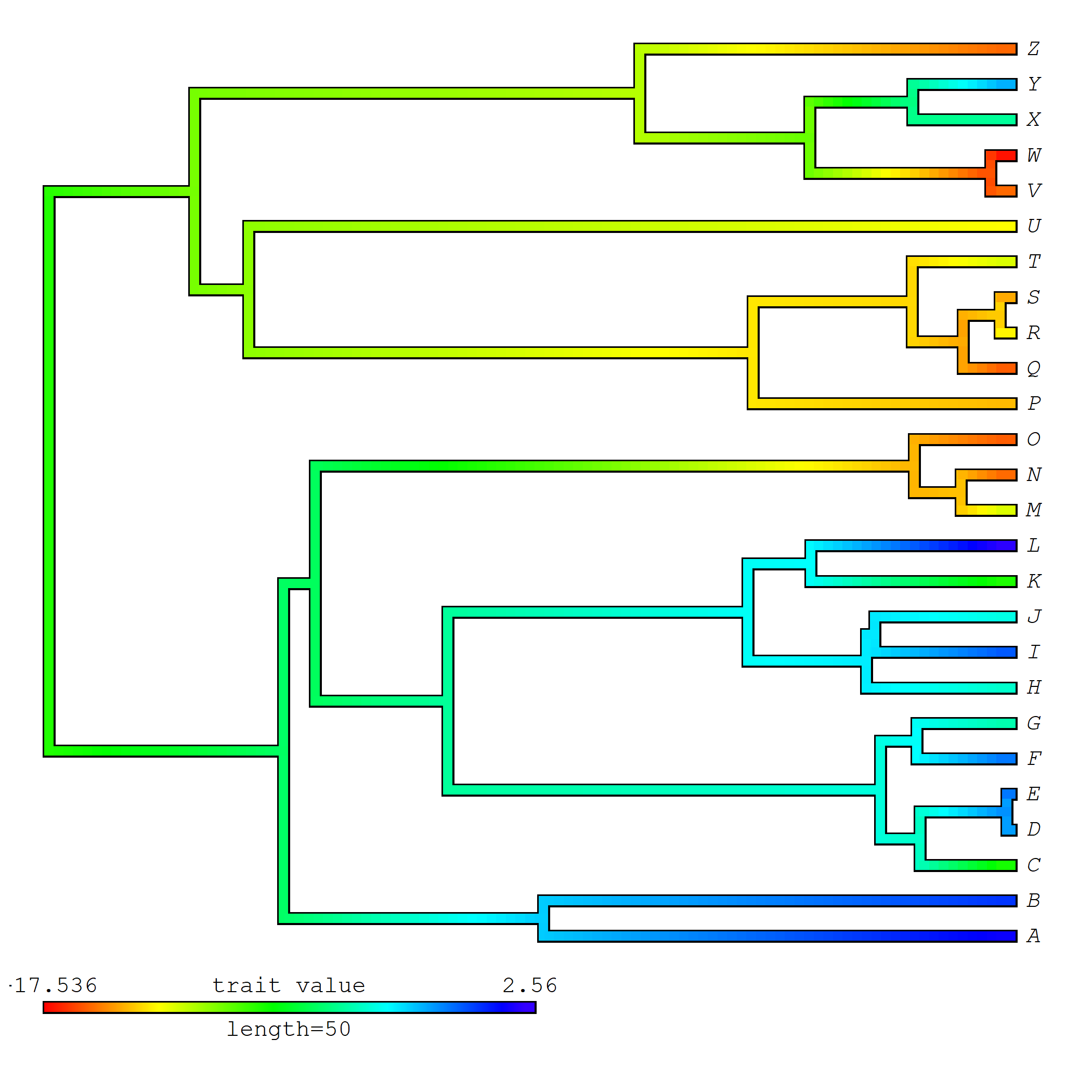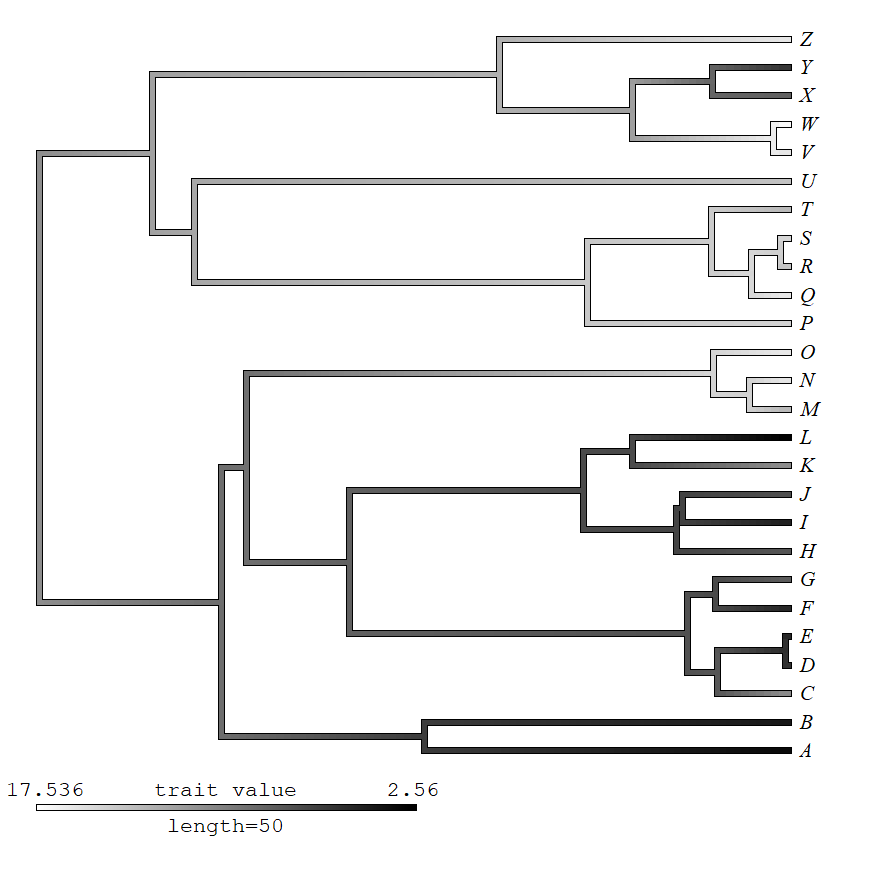## Wednesday, May 15, 2019

### Using non-default fonts when plotting trees in R

A recent comment on an old post asked of a `contMap` or `densityMap` plot:

'Is there a way to use serif fonts for the color bar and cladelabels?'

The answer is 'yes' - and there are a few different ways to do it. Unfortunately, there is presently no way to automatically use different font styles for different attributes of the `contMap` plot; however, there are some work-arounds, as I'll illustrate below.

First, let's consider the simplest case of plotting to the R graphical user interface in an interactive R session and in which we want to use a single font style (let's say `'serif'`) for all the text on a plot.

We can do this most easily by setting the value of the graphical parameter `family` to the desired font. Note that readers should review the help page for `par` to find out which fonts are supported:

``````library(phytools)
## simulate some data for the purposes of illustration
tree<-pbtree(n=26,tip.label=LETTERS,
scale=100)
x<-fastBM(tree)
## create our 'contMap' object
obj<-contMap(tree,x,plot=FALSE)
## set our font family
par(family="serif")
## plot
plot(obj,fsize=1.2,lwd=6)
``````The same thing can be done if we want to export our plots as a PDF or PNG; except that we have to keep in mind that our fonts will differ depending on what type of file we choose. For instance:

``````png(file="contMap-tree.png",width=7,height=7,
units="in",res=300,family="mono")
plot(obj,fsize=0.9,lwd=6)
dev.off()
``````
``````## windows
##       2
``````

Which will look like this:If we want to use different fonts in (for instance) the tip labels & the legend, this gets a bit more complicated. The only way I can think to do this is to plot the object absent certain elements, and then add these in aferwards. Say, for instance:

``````plotTree(obj\$tree,plot=FALSE)
xlim<-get("last_plot.phylo",envir=.PlotPhyloEnv)\$x.lim
par(family="mono")
plot(setMap(obj,c("white","black")),ftype="off",xlim=xlim)
par(family="serif")
tiplabels(obj\$tree\$tip.label,tip=1:Ntip(obj\$tree),pos=4,
font=3,frame="none")
``````(The first step is so that I can get the necessary x dimension to permit size for the tip labels even though I don't plan to plot the labels.)

Similarly, we could plot our `"contMap"` object with the labels & then add our gradient legend afterwards using `add.color.bar`.

That's all there is to it.